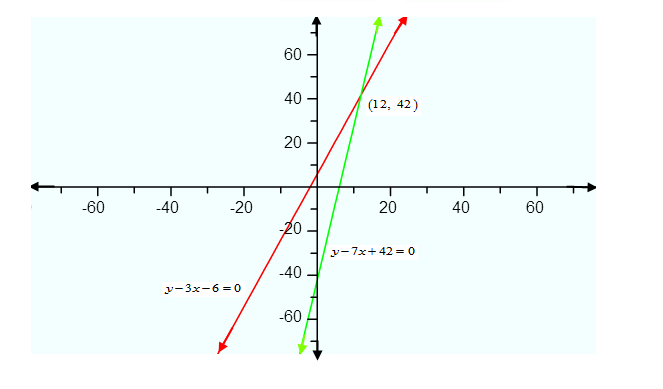# Aftab tells his daughter,`
Question:

Aftab tells his daughter, "Seven years ago, I was seven times as old as you were then. Also, three years from now, I shall be three times as old as you will be". Is not this interesting? Represent this situation algebraically and graphically.

Solution:

Let age of Aftab is $x$ years and age of his daughter is $y$ years. 7 Years ago his age was 7 times older as her daughter was. Then

$\Rightarrow y-7=7(x-7)$

$\Rightarrow y-7=7 x-49$

$\Rightarrow y-7 x+42=0$ ...(1)

Three years from now, he will be three times older as his daughter will be, then

$\Rightarrow y+3=3(x+3)$

$\Rightarrow y+3=3 x+9$

$\Rightarrow y-3 x-6=0$ $.(2)$

Hence the algebraic representation are $y-3 x-6=0$ and $y-7 x+42=0$